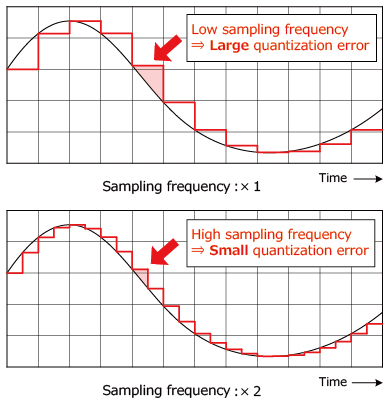# ADC ＜Basic A/D Converter Configurations＞

Numerous configurations exist for ADC (A/D converter). We cover the basic configurations below.

## Flash Method

This type of A/D converter utilizes 2N-1 comparators (for an N bit converter) to compare the analog signal with successive reference voltages. The results are then converted into digital format using an encoder.

Features:

• Analog signals are converted into digital signals directly (since the comparators themselves are the sampling devices), making a Sample and Hold circuit unnecessary.
• This allows for extremely fast conversion (with sampling frequencies above 1GHz possible).
• However, the relatively larger size and power consumption (due to the number of comparators are required: 2N-1) limit resolution to around 8bits.## Pipeline Method

In the case of a 1.5bit/stage configuration, the following processes are repeated in order from Stage 1 that determines MSB via pipeline operation (VREF: Reference Voltage).

• At the same time the analog input is converted by an A/D converter into a 3-value digital format (1.5bit). (Here the digital output stage is defined.)
• These digital values are then converted into analog values using a digital to analog converter (DAC).
• D="00" → DAC output：-VREF/2
• D="01" → DAC output：0
• D="10" → DAC output：+VREF/2
• The negative DAC output voltage is amplified (x2) and output to the next stage.

Once processing of Stage N that determines LSB is completed, the delay between each stage is corrected then digital conversion completed by adding the respective digital output.Characteristics:

• High resolution enabled (up to 16bit)
• High-speed conversion possible (200MHz max. sampling frequency)
• There is a necessary wait time until the digital signal is output (based on bipolar operation), making this impractical for applications requiring real-time processing (i.e. control).

## Approximation Method

This method compares the sampled analog input with the converter's output in succession, starting with the MSB.

• A successive approximation register (SAR), which is designed to supply an approximate digital code to the internal DAC, is initialized so that the most significant bit (MSB) is set to '1'.
• The digital values from the SAR are converted into equivalent analog values by the internal DAC.
• The sampled input voltage is compared with the DAC output voltage.
• If the sampled voltage > DAC output voltage → MSB = 1
• If the sampled voltage < DAC output voltage → MSB = 0

The digital conversion is completed by repeating the operation up to LSB.Characteristics:

• High resolution conversion possible (up to 18bit)
• Since a clock cycle is required (resolution + α), conversion speed is moderate (10MHz max. sampling frequency)
• Good response. Connecting a multiplexer to the the input makes it easy to switch analog signals.

## ΔΣ Method

After oversampling of the analog signal is performed and converted into a series of pulses corresponding to the amplitudes of the analog signal through ΔΣ modulation, conversion into digital signals at the original sampling rate is completed by filtering data and removing out-of-band noise using a digital filter.

### Oversampling

Quantization error is decreased by sampling at a higher frequency than the original sampling frequency.### ΔΣ Modulation

The difference (Δ) between the DAC output voltage and sampled voltage (via oversampling) is summed using an integrator. The sampled values are then converted into a series of pulses by comparing with a reference voltage using a comparator.

The output pulses are fed back to the input (which is delayed by one sampling operation), reducing the quantization error generated at the comparator in the low-frequency region. This requires modulation in order to become larger at higher frequencies.The pulse series output from the ΔΣ modulator often has a large quantization error component in the high-frequency region in addition to the original signal component. However, since these components are separated in frequency, it is easy to remove only the quantization error component using a digital filter and make it possible to achieve higher resolution compared with other methods.

Characteristics:

• Among the highest resolution of all A/D converters (up to 24bit)
• Relatively slow conversion speed (200kHz max. sampling frequency)
• Poor response makes it unsuitable for applications that connect a multiplexer to the input for switching between analog signal sources.